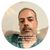# New to Qlik Sense

If you’re new to Qlik Sense, start with this Discussion Board and get up-to-speed quickly.

Announcements
QlikWorld 2023, a live, in-person thrill ride. Save \$300 before February 6: REGISTER NOW!
cancel
Showing results for
Did you mean:Contributor II

## Aggr fonction with sliders variables

Hello,

I am trying to create a dynamic dimension bases on variable sliders.

For example I have a Product table with several variables such as Size, Cost, Weight... and I would like to show the products corresponding to the selected values variables.

Each variables has a value define by a slider.

So I try to use a Aggr fonction with those variables but I can't manage to make them independent.

``````=aggr(ONLY({<Size={"<=\$(=V_Size)"},Cost={"<=\$(=V_Cost)"}>}Product),Product)
``````

The code use doesn't work, if the value of the variable Size is equal to 0 and I am trying to increase the value of the variable Cost with a Slider, it return none products.

So i don't know how I can return Products whose criterion corresponds to one of the two variables or both.

I tried several solutions by concatenating adding, multiplying each aggregation but it doesn't work.

Can you help me to solve this issue.

Thank you.

Labels (3)

• ### Variables

2 RepliesMVP

Probably you don't need '=' sign after '\$' as you don't have any function used in the variable, not sure if I understood it properly..

``aggr(ONLY({<Size={"<=\$(V_Size)"},Cost={"<=\$(V_Cost)"}>}Product),Product)``MVP & Luminary

If I understand it right you want to have a OR connection between your conditions and not an AND. If so you may try it in this way:

``=aggr(ONLY({<Size={"<=\$(=V_Size)"}> + < Cost={"<=\$(=V_Cost)"}>}Product),Product)``

- MarcusTags
Community Browser